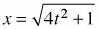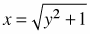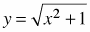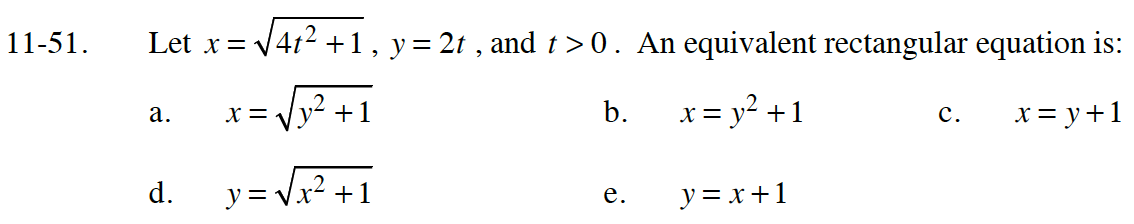### Home > CALC > Chapter Ch11 > Lesson 11.2.1 > Problem11-51

11-51.
1. Let, y = 2t, and t > 0. An equivalent rectangular equation is: Homework Help ✎

1.2. x = y2 + 1

3. x = y + 1

4.5. y = x + 1